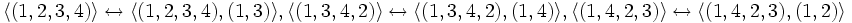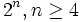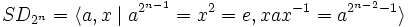# Cyclic maximal subgroup of dihedral group:D8

(Redirected from Z4 in D8)
This article is about a particular subgroup in a group, up to equivalence of subgroups (i.e., an isomorphism of groups that induces the corresponding isomorphism of subgroups). The subgroup is (up to isomorphism) cyclic group:Z4 and the group is (up to isomorphism) dihedral group:D8 (see subgroup structure of dihedral group:D8).
The subgroup is a normal subgroup and the quotient group is isomorphic to cyclic group:Z2.
VIEW: Group-subgroup pairs with the same subgroup part | Group-subgroup pairs with the same group part| Group-subgroup pairs with the same quotient part | All pages on particular subgroups in groups

This article discusses the dihedral group of order eight and the cyclic maximal subgroup (the subgroup of order four) of this group. We call the dihedral group$G$, and use the following presentation:$\! G := \langle a,x \mid a^4 = x^2 = e, xax = a^{-1} \rangle$

and we set:$\! H = \langle a \rangle$.

In the typical description of$G$ as a permutation group:$\! G := \langle (1,2,3,4), (1,3) \rangle$

we have:$\! H = \langle (1,2,3,4) \rangle$

## Cosets

The subgroup has index two and is hence a normal subgroup. Its left cosets coincide with its right cosets, and there are exactly two of them: the subgroup itself and the rest of the group:$H = \{ e, a, a^2, a^3 \}, G \setminus H = \{ x, ax, a^2x, a^3x \}$

## Complements

COMPLEMENTS TO NORMAL SUBGROUP: TERMS/FACTS TO CHECK AGAINST:
TERMS: permutable complements | permutably complemented subgroup | lattice-complemented subgroup | complemented normal subgroup (normal subgroup that has permutable complement, equivalently, that has lattice complement) | retract (subgroup having a normal complement)
FACTS: complement to normal subgroup is isomorphic to quotient | complements to abelian normal subgroup are automorphic | complements to normal subgroup need not be automorphic | Schur-Zassenhaus theorem (two parts: normal Hall implies permutably complemented and Hall retract implies order-conjugate)$H$ as four permutable complements, which are also precisely its lattice complements, and none of them is a normal complement.

### Properties related to complementation

Property Meaning Satisfied? Explanation Comment
complemented normal subgroup normal subgroup with permutable complement Yes see above
permutably complemented subgroup subgroup with permutable complement Yes (via complemented normal)
lattice-complemented subgroup subgroup with lattice complement Yes (via permutably complemented)
retract has a normal complement No
direct factor normal subgroup with normal complement No

## Arithmetic functions

Function Value Explanation
order of whole group 8
order of subgroup 4
index 2
size of conjugacy class 1

## Effect of subgroup operators

Function Value as subgroup (descriptive) Value as subgroup (link) Value as group
normalizer whole group -- dihedral group:D8
centralizer$\langle a \rangle$ -- the subgroup itself current page cyclic group:Z4
normal core the subgroup itself current page cyclic group:Z4
normal closure the subgroup itself current page cyclic group:Z4
characteristic core the subgroup itself current page cyclic group:Z4
characteristic closure the subgroup itself current page cyclic group:Z4

## Related subgroups

### Intermediate subgroups

There are no properly intermediate subgroups because the subgroup is a maximal subgroup.

### Smaller subgroups

Value of smaller subgroup (descriptive) Isomorphism class of smaller subgroup Smaller subgroup in subgroup Smaller subgroup in whole group$\langle a^2 \rangle$ cyclic group:Z2 Z2 in Z4 center of dihedral group:D8

### Relation with other subgroups

Other subgroup by generators Other subgroup by link Intersection value Join value$\langle a^2, x \rangle$ Klein four-subgroups of dihedral group:D8$\langle a^2 \rangle$ (center of dihedral group:D8) whole group$\langle a^2, ax \rangle$ Klein four-subgroups of dihedral group:D8$\langle a^2 \rangle$ (center of dihedral group:D8) whole group

## Bigger groups

### Groups containing the ambient group

Isomorphism class of bigger group Group in bigger group Subgroup in bigger group
symmetric group:S4 D8 in S4 Z4 in S4

## Subgroup properties

### Invariance under automorphisms and endomorphisms: basic properties

Property Meaning Satisfied? Explanation Comment
normal subgroup invariant under inner automorphisms Yes nilpotent implies every maximal subgroup is normal, also characteristic implies normal quotient group is cyclic of order two
characteristic subgroup invariant under all automorphisms Yes only cyclic subgroup of order four
fully invariant subgroup invariant under all endomorphisms No retraction with kernel one of the Klein four-subgroups of dihedral group:D8 and image a subgroup of order two generated by a reflection does not preserve this subgroup See endomorphism structure of dihedral group:D8

### Invariance under automorphisms and endomorphisms: advanced properties

Property Meaning Satisfied? Explanation Comment
retraction-invariant subgroup invariant under all retractions, i.e., idempotent endomorphisms No retraction with kernel one of the Klein four-subgroups of dihedral group:D8 and image a subgroup of order two generated by a reflection does not preserve this subgroup See endomorphism structure of dihedral group:D8
normality-preserving endomorphism-invariant subgroup invariant under all normality-preserving endomorphisms Yes
finite direct power-closed characteristic subgroup any finite direct power of subgroup is characteristic in corresponding finite direct power of whole group Yes normality-preserving endomorphism-invariant implies finite direct power-closed characteristic
1-automorphism-invariant subgroup invariant under 1-automorphisms Yes A 1-automorphism restricts to an isomorphism on cyclic subgroups, and this is the only cyclic subgroup of order four.
quasiautomorphism-invariant subgroup invariant under quasiautomorphisms Yes Follows from being 1-automorphism-invariant.
1-endomorphism-invariant subgroup invariant under all 1-endomorphisms No Follows from not being fully invariant

### Advanced properties related to resemblance and corollaries for invariance

Property Meaning Satisfied? Explanation Comment
isomorph-free subgroup no other isomorphic subgroup Yes clear from subgroup structure of dihedral group:D8
isomorph-containing subgroup contains all isomorphic subgroups Yes isomorph-free equals isomorph-containing for finite subgroups
prehomomorph-contained subgroup for any surjective homomorphism$\varphi:K \to H$ for$K \le G$, we have$H \le K$ Yes
homomorph-containing subgroup contains all homomorphic images No (via fully invariant)
normal-homomorph-containing subgroup contains all homomorphic images that are normal subgroups Yes
maximal characteristic subgroup Yes
intermediately characteristic subgroup Yes
image-closed characteristic subgroup For any surjective homomorphism$\varphi:G \to K$,$\varphi(H)$ is characteristic in$K$ No The quotient map by the center is a counterexample characteristicity does not satisfy image condition -- this is a counterexample of smallest order
transfer-closed characteristic subgroup Intersection with any subgroup is characteristic in that subgroup No Intersection with$\langle a^2, x \rangle$ is not characteristic in it characteristicity does not satisfy transfer condition

### Properties related to embeddings in bigger groups

Property Meaning Satisfied? Explanation Comment
isomorph-normal subgroup every isomorphic subgroup is normal Yes because it is isomorph-free
coprime automorphism-invariant subgroup invariant under all automorphisms of coprime order Yes because it is characteristic; also, no non-identity automorphisms of coprime order.
fusion system-relatively weakly closed subgroup weakly closed subgroup for any fusion system on the whole group Yes isomorph-normal coprime automorphism-invariant implies fusion system-relatively weakly closed
Sylow-relatively weakly closed subgroup weakly closed subgroup whenever we embed dihedral group:D8 as a Sylow subgroup in a bigger group Yes isomorph-normal coprime automorphism-invariant implies Sylow-relatively weakly closed
finite-pi-potentially fully invariant subgroup there is a finite 2-group containing$G$ in which$H$ is fully invariant Yes homocyclic normal implies finite-pi-potentially fully invariant in finite
finite-pi-potentially characteristic subgroup there is a finite 2-group containing$G$ in which$H$ is characteristic Yes (via finite-pi-potentially fully invariant)
potentially fully invariant subgroup there is a group containing$G$ in which$H$ is fully invariant Yes (via finite-pi-potentially fully invariant)

### Properties related to conjugacy and splitting and transitivity of normality

Property Meaning Satisfied? Explanation
central factor product with its centralizer is the whole group No The subgroup is a self-centralizing subgroup
conjugacy-closed subgroup any two elements of the subgroup conjugate in the whole group are conjugate in the subgroup No The elements$a, a^3$ are conjugate in the whole group but not in the subgroup.
transitively normal subgroup every normal subgroup of the subgroup is normal in the whole group Yes cyclic normal implies transitively normal
CEP-subgroup every congruence on the subgroup extends to a congruence on the whole group Yes Follows from being transitively normal.

### Generic maximality notions

Property Meaning Satisfied? Explanation Comment
maximal subgroup proper subgroup not contained in any other proper subgroup Yes Hence also a maximal subgroup of group of prime power order
subgroup of prime index (here, subgroup of index two) index is a prime number Yes In a group of prime power order, equivalent to being a maximal subgroup
maximal normal subgroup proper normal subgroup with simple quotient Yes In a group of prime power order, equivalent to being a maximal subgroup
isomorph-normal subgroup every isomorphic subgroup is normal Yes Any maximal subgroup of group of prime power order satisfies this property
order-normal subgroup every subgroup of the same order is normal Yes Any maximal subgroup of group of prime power order satisfies this property

### Maximality notions related to abelianness

Property Meaning Satisfied? Explanation Comment
abelian characteristic subgroup abelian as well as characteristic Yes
maximal among abelian characteristic subgroups abelian characteristic, not contained in bigger abelian characteristic subgroup Yes In fact, it is the only subgroup that is maximal among abelian characteristic subgroups.
critical subgroup characteristic, self-centralizing, commutator-in-center subgroup, and Frattini-in-center group Yes
constructibly critical subgroup critical subgroup whose center is maximal among abelian characteristic subgroups Yes In fact, it is the only constructibly critical subgroup.
abelian subgroup of maximum order abelian subgroup whose order is the largest among orders of abelian subgroups Yes there are two others, the Klein four-subgroups of dihedral group:D8
maximal among abelian subgroups abelian subgroup not contained in any other abelian subgroup Yes there are two others, the Klein four-subgroups of dihedral group:D8
subgroup with abelianization of maximum order abelianization has maximum order among all subgroups Yes there are three others, the Klein four-subgroups of dihedral group:D8, and the whole group
abelian subgroup of maximum rank abelian subgroup whose rank (number of generators) is largest No Klein four-subgroups of dihedral group:D8 have larger rank
centrally large subgroup product of size and center's size is largest Yes others are Klein four-subgroups of dihedral group:D8 and whole group
centralizer-large subgroup product of size and centralizer's size is largest Yes others are center of dihedral group:D8, Klein four-subgroups of dihedral group:D8, and whole group

## In the context of different ways of thinking about the dihedral group

### As the group of symmetries of a square

If we think of the dihedral group of order eight as the group of symmetries of the square, the cyclic maximal subgroup is the subgroup comprising the rotations, or the orientation-preserving symmetries.

Related interpretations:

• The dihedral group has a two-dimensional faithful irreducible representation over any field of characteristic not equal to two (see linear representation theory of dihedral group:D8). This representation can be realized as taking values in the orthogonal group. This representation is essentially a generalization of the action on the square. Under any such representation, the cyclic maximal subgroup comprises the elements of determinant one, which are the rotations.
• When the field has size$3$ or$5$, this representation surjects onto the orthogonal group. In other words,$O(2,3)$ and$O(2,5)$ are both isomorphic to the dihedral group. In this case, the cyclic maximal subgroup is$SO(2,3)$ or$SO(2,5)$.

## In the context of different embeddings of the dihedral group

Since the cyclic maximal subgroup is an isomorph-normal characteristic subgroup, it is in particular an isomorph-normal coprime automorphism-invariant subgroup, and thus a fusion system-relatively weakly closed subgroup and thus a Sylow-relatively weakly closed subgroup. In other words, for any group containing the dihedral group of order eight as a$2$-Sylow subgroup, this subgroup is a weakly closed subgroup. Thus, there is a bijection between the set of$2$-Sylow subgroups of such a group and the set of cyclic subgroups of order four.

### Inside the symmetric group of degree four

The dihedral group of order eight is the$2$-Sylow subgroup inside the symmetric group of degree four. There are three$2$-Sylow subgroups, and these correspond to the three cyclic subgroups of order four. Namely:$\langle (1,2,3,4) \rangle \leftrightarrow \langle (1,2,3,4), (1,3) \rangle, \langle (1,3,4,2) \rangle \leftrightarrow \langle (1,3,4,2), (1,4) \rangle, \langle (1,4,2,3) \rangle \leftrightarrow \langle (1,4,2,3), (1,2) \rangle$.

The Sylow subgroup in each case is the normalizer of the corresponding cyclic maximal subgroup.

### Inside the projective special linear group$PSL(3,2)$

The dihedral group of order eight is a 2-Sylow subgroup inside this group as well. PLACEHOLDER FOR INFORMATION TO BE FILLED IN: [SHOW MORE]

## Comparison with cyclic maximal subgroup in other dihedral groups

Further information: Cyclic subgroup is characteristic in dihedral group

In any dihedral group, given by:$D_{2n} = \langle a,x \mid a^n = x^2 = e, xax = a^{-1} \rangle$.

For$n \ge 3$, the cyclic subgroup$\langle a \rangle$ is a characteristic subgroup. However, for all$n \ne 4$, the cyclic subgroup can be characterized as the centralizer of commutator subgroup. But, in the case$n = 4$ (which is the one we're considering here), the cyclic maximal subgroup is not the centralizer of commutator subgroup.

Here are some of the salient differences between the cyclic maximal subgroup in the dihedral group of order eight and the cyclic maximal subgroup in the dihedral groups of order$2^n, n \ge 4$:

• In the dihedral group of order eight, the cyclic maximal subgroup is not the centralizer of derived subgroup. In dihedral groups of order$2^n, n \ge 4$, it is the centralizer of derived subgroup.
• In the dihedral group of order eight, the cyclic maximal subgroup is an abelian subgroup of maximum order, but it is not the only one. In dihedral groups of order$2^n, n \ge 4$, it is the unique abelian subgroup of maximum order. In particular, in the higher cases, the cyclic maximal subgroup equals the join of abelian subgroups of maximum order (called the Thompson subgroup) and hence also equals the ZJ-subgroup (the center of the join of abelian subgroups of maximum order).
• In the dihedral group of order eight, the cyclic maximal subgroup is one out of four different centrally large subgroups. In dihedral groups of order$2^n, n \ge 4$, it is the unique centrally large subgroup.
• In the dihedral group of order eight, the cyclic maximal subgroup is one of four subgroups with abelianization of maximum order. In dihedral groups of order$2^n, n \ge 4$, it is the unique subgroup with abelianization of maximum order.

### Comparison with cyclic maximal subgroup in other semidihedral groups

We define, for$n \ge 4$:$SD_{2^n} = \langle a,x \mid a^{2^{n-1}} = x^2 = e, xax^{-1} = a^{2^{n-2} - 1} \rangle$.

### Comparison with the$M_{2^n}$-groups

We define, for$n \ge 3$:$M_{2^n} = \langle a,x \mid a^{2^{n-1}} = x^2 = e, xax^{-1} = a^{2^{n-2} + 1} \rangle$.

Suppose we know that$G$ is a group isomorphic to dihedral group:D8. Then, we can define the subgroup$H$ as follows:
H := Filtered(NormalSubgroups(G),K -> IdGroup(K) = [4,1]);